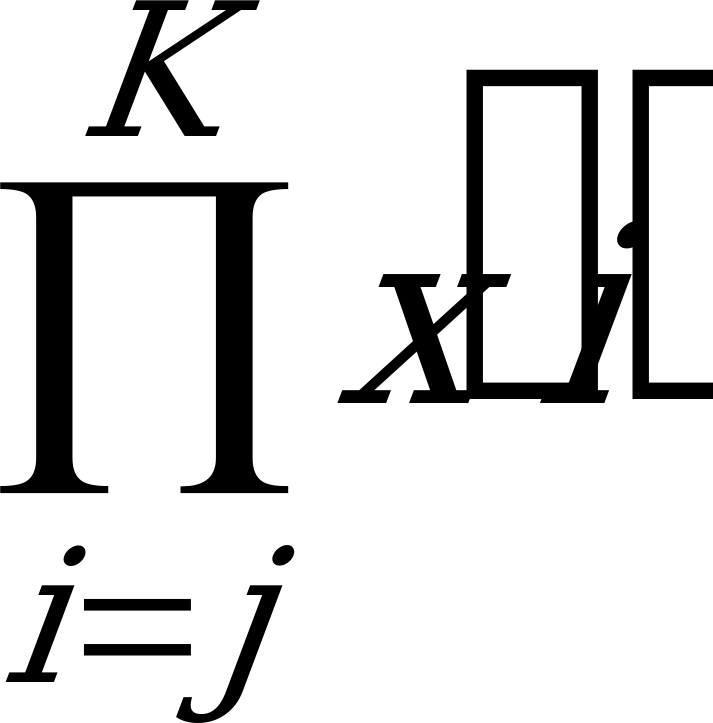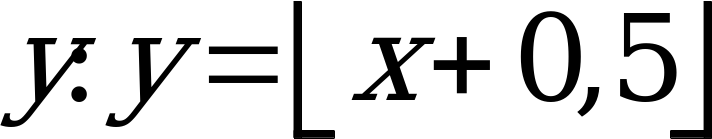## 3 Definitions, symbols and abbreviations

06.203GPPHalf rate speech transcodingTS

## 3.1 Definitions

For the purposes of this ETS, the following definitions apply:

adaptive codebook: The adaptive codebook is derived from the long term filter state. The lag value can be viewed as an index into the adaptive codebook.

adaptive pitch prefilter: In the GSM half rate speech decoder, this filter is applied to the excitation signal to enhance the periodicity of the reconstructed speech. Note that this is done prior to the application of the short term filter.

adaptive spectral postfilter: In the GSM half rate speech decoder, this filter is applied to the output of the short term filter to enhance the perceptual quality of the reconstructed speech.

allowable lags: The set of lag values which may be coded by the GSM half rate speech encoder and transmitted to the GSM half rate speech decoder. This set contains both integer and fractional values (see table 3).

analysis window: For each frame, the short term filter coefficients are computed using the high pass filtered speech samples within the analysis window. The analysis window is 170 samples in length, and is centred about the last 100 samples in the frame.

basis vectors: A set of M, M1, or M2 vectors of length Ns used to generate the VSELP codebook vectors. These vectors are not necessarily orthogonal.

closed loop lag search: A process of determining the near optimal lag value from the weighted input speech and the long term filter state.

closed loop lag trajectory: For a given frame, the sequence of near optimal lag values whose elements correspond to each of the four subframes as determined by the closed loop lag search.

codebook: A set of vectors used in a vector quantizer.

codeword (OR Code): An M, M1, or M2 bit symbol indicating the vector to be selected from a VSELP codebook.

delta (LAG) code: A four bit code indicating the change in lag value for a subframe relative to the previous subframe’s coded lag. For frames in which the long term predictor is enabled (MODE 1, 2, or 3), the lag for subframe 1 is independently coded using eight bits, and delta codes are used for subframes 2, 3, and 4.

direct form coefficients: One of the formats for storing the short term filter parameters. All filters which are used to modify speech samples use direct form coefficients.

fractional lags: A set of lag values having sub-sample resolution. Note that not every fractional lag value considered in the GSM half rate speech encoder is an allowable lag value.

frame: A time interval equal to 20 ms, or 160 samples at an 8 kHz sampling rate.

harmonic noise weighting filter: This filter exploits the noise masking properties of the spectral peaks which occur at harmonics of the pitch frequency by weighting the residual error less in regions near the pitch harmonics and more in regions away from them. Note that this filter is only used when the long term filter is enabled (MODE = 1, 2 or 3).

high pass filter: This filter is used to de-emphasize the low frequency components of the input speech signal.

integer lags: A set of lag values having whole sample resolution.

interpolating filter: An FIR filter used to estimate sub-sample resolution samples, given an input sampled with integer sample resolution.

lag: The long term filter delay. This is typically the pitch period, or a multiple or sub-multiple of it.

long term filter: This filter is used to generate the periodic component in the excitation for the current subframe. This filter is only enabled for MODE = 1, 2 or 3.

LPC coefficients: Linear Predictive Coding (LPC) coefficients is a generic descriptive term for describing the short term filter coefficients.

open loop lag search: A process of estimating the near optimal lag directly from the weighted speech input. This is done to narrow the range of lag values over which the closed loop lag search shall be performed.

open loop lag trajectory: For a given frame, the sequence of near optimal lag values whose elements correspond to the four subframes as determined by the open loop lag search.

reflection coefficients: An alternative representation of the information contained in the short term filter parameters.

residual: The output signal resulting from an inverse filtering operation.

short term filter: This filter introduces, into the excitation signal, short term correlation which models the impulse response of the vocal tract.

soft interpolation: A process wherein a decision is made for each frame to use either interpolated or uninterpolated short term filter parameters for the four subframes in that frame.

soft interpolation bit: A one bit code indicating whether or not interpolation of the short term parameters is to be used in the current frame.

spectral noise weighting filter: This filter exploits the noise masking properties of the formants (vocal tract resonances) by weighting the residual error less in regions near the formant frequencies and more in regions away from them.

subframe: A time interval equal to 5 ms, or 40 samples at an 8 kHz sampling rate.

vector quantization: A method of grouping several parameters into a vector and quantizing them simultaneously.

GSP0 vector quantizer: The process of vector quantization, its intermediate parameters (GS and P0) for the coding of the excitation gains b and g.

VSELP codebook: Vector-Sum Excited Linear Predictive (VSELP) codebook, used in the GSM half rate speech coder, wherein each codebook vector is constructed as a linear combination of the fixed basis vectors.

zero input response: The output of a filter due to all past inputs, i.e. due to the present state of the filter, given that an input of zeros is applied.

zero state response: The output of a filter due to the present input, given that no past inputs have been applied, i.e. given the state information in the filter is all zeroes.

## 3.2 Symbols

For the purposes of this ETS, the following symbols apply:

A(z) Short term spectral filter.

ai The LPC coefficients.

bL(n) The output of the long term filter state (adaptive codebook) for lag L.

 The long term filter coefficient.

C(z) Second weighting filter.

e(n) Weighted error signal

fj(i) The coefficients of the jth phase of the 10th order interpolating filter used to evaluate candidate fractional lag values; i ranges from 0 to Pf‑1.

gj(i) The coefficients of the jth phase of the 6th order interpolating filter used to interpolate C’s and G’s as well as fractional lags in the harmonic noise weighting; i ranges from 0 to Pg‑1.

 The gain applied to the vector(s) selected from the VSELP codebook(s).

H A M2 bit code indicating the vector to be selected from the second VSELP codebook (when operating in mode 0).

I A M or M1 bit code indicating the vector to be selected from one of the two first VSELP codebooks.

L The long term filter lag value.

Lmax 142 (samples), the maximum possible value for the long term filter lag.

Lmin 21 (samples), the minimum possible value for the long term filter lag.

M 9, the number of basis vectors, and the number of bits in a codeword, for the VSELP codebook used in modes 1, 2, and 3.

M1 7, the number of basis vectors, and the number of bits in a codeword, for the first VSELP codebook used in mode 0.

M2 7, the number of basis vectors, and the number of bits in a codeword, for the second VSELP codebook used in mode 0.

MODE A two bit code indicating the mode for the current frame (see annex A).

NA 170, the length of the analysis window. This is the number of high pass filtered speech samples used to compute the short term filter parameters for each frame.

NF 160, the number of samples per frame (at a sampling rate of 8 kHz).

Np 10, the short term filter order.

Ns 40, the number of samples per subframe (at a sampling rate of 8 kHz).

P1 6, the number of bits in the prequantizer for the r1 – r3 vector quantizer.

P2 5, the number of bits in the prequantizer for the r4 – r6 vector quantizer.

P3 4, the number of bits in the prequantizer for the r7 – r10 vector quantizer.

Pf The order of one phase of an interpolating filter used to evaluate candidate fractional lag values. Pf equals 10 for j ¹ 0 and equal to 1 for j = 0.

Pg The order of one phase of an interpolating filter, fj(n), used to interpolate C’s and G’s as well as fractional lags in the harmonic noise weighting, Pg equals 6.

pitch The time duration between the glottal pulses which result when the vocal chords vibrate during speech production.

Q1 11, the number of bits in the r1 – r3 reflection coefficient vector quantizer.

Q2 9, the number of bits in the r4 – r6 reflection coefficient vector quantizer.

Q3 8, the number of bits in the r7 – r10 reflection coefficient vector quantizer.

R0 A five bit code used to indicate the energy level in the current frame.

r(n) The long term filter state (the history of the excitation signal); n < 0

rL(n) The long term filter state with the adaptive codebook output for lag L appended.

s'(n) Synthesized speech.

W(z) Spectral weighting filter.

hnw The harmonic noise weighting filter coefficient.

 The adaptive pitch prefilter coefficient.

éxù Ceiling function: the largest integer y where y < x + 1,0.

ëxû Floor function: the largest integer y where y £ x.

Summation: x(j)+x(j+1)+…+x(K).Product: x(j)(x(j+1))….(x(K)).

max(x,y) Find the larger of two numbers x and y.

min(x,y) Find the smaller of two numbers x and y.

round(x) Round the non-integer x to the closest integery: y=x+0,5.

## 3.3 Abbreviations

For the purposes of this ETS, the following abbreviations apply:

AFLAT Autocorrelation Fixed point LAttice Technique

CELP Code Excited Linear Prediction

FLAT Fixed Point Lattice Technique

LTP Long Term Predictor

SST Spectral Smoothing Technique

VSELP Vector-Sum Excited Linear Prediction